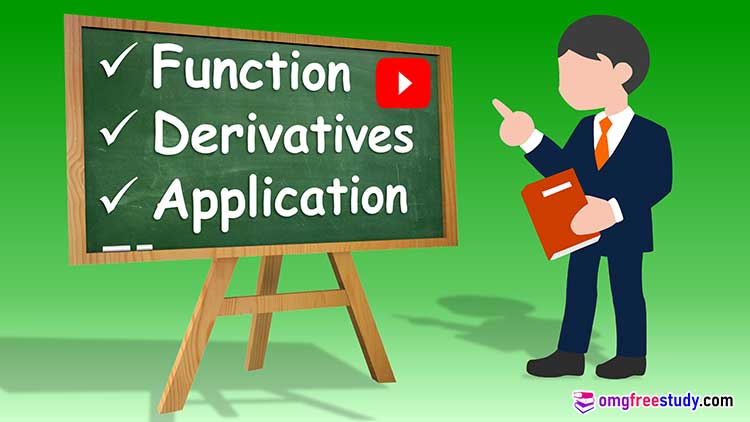# Differential Calculus | Functions | Derivative & its Application

On this page we are trying to present to you a set of Differential Calculus questions in PDF format. Along with this, the answers of this questions in the question set have been uploaded on YouTube.## Functions ( Differential Calculus ):

Concept of function and simple examples.

Definition:

If A and B are two non-empty sets, such that every elements of the set A is related to one and only one element or set B, then relation is called as function. Functions are denoted by any alphabetic.

Generally we denoted by f. suppose there are two variables x and y. If the variable y is related to the variable x such that for a given value of x in its interval we are able to determine the corresponding value of y, then we say that y is function of x. Since x can take any value of its interval. It is called independent variable and value of y depends on value of x is called dependent variable.

Value of Functions ( Differential Calculus ):

If y = f(x) is a given function then the value of f (x) obtained by replacing ‘x’ by ‘a’ is called the value of the function.

To find value of particular function consider one example

F(x) = is a function at particular point ‘x’

We can put the value of ‘x’ in place of function.

After solving some steps we can get the value of Function

For detail Solution of Function Numerical we can join with us on our official YouTube Channel ‘Open Mind Guruji’ or watch below video link.

### Odd and Even Function

To identify odd and even function only we have to put (-x) in place of (x) only. ( Differential Calculus )

If f(-x)=-f(x) in solution then the given function is called as Odd  function

If f(-x)= f(x) in solution then the given function is called as Even function.

For better understanding watch below video Lectures.

Lecture 1

Lecture 2

Lecture 3

Lecture 4

Lecture 5

## Derivatives :

Topic of Differential Calculus

Rules of derivatives such as sum, product, quotient of functions.

Derivative of composite functions (chain Rule), implicit and parametric functions.

Derivatives of inverse, logarithmic and exponential functions.

For better understanding watch below video Lectures.

Lecture 1

Lecture 2

Lecture 3

Lecture 4

## Applications of derivative:

Topic of Differential Calculus

• Second order derivative
• Equation of tangent and normal
• Maxima and minima